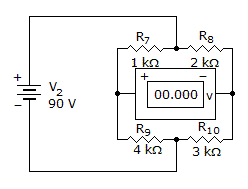# Electronics - Series-Parallel Circuits - Discussion

### Discussion :: Series-Parallel Circuits - True or False (Q.No.5)

5.

The voltmeter will read 18 volts.[A]. True [B]. False

Explanation:

No answer description available for this question.

 Shreya said: (Dec 31, 2013) Lets say point between R8 and R10 be A and point between R7 and R9 be B. Now, R8, R10 in series so Rt1 = 2+3 = 5 ohm. And R7, R9 in series so Rt2 = 1+4 = 5 ohm. Rt=(Rt1Rt2)/(Rt1+Rt2) = 5/2 ohm. Total current = V/Rt = (90*2)/5 = 36 amp. Since Rt1 and Rt2 are equal therefore equal current will flow through each branch = 36/2 = 18 amp. Voltage drop across R8 = 18*2 = 36v. Potential at point A = 90-36 = 54v. Voltage drop across R7 = 18*1 = 18v. Potential at point B = 90-18 = 72v. Potential diff. between A and B gives voltmeter reading = 72-54 = 18v.

 Kiran V said: (Jan 16, 2017) Good explanation @Shreya.